### IMO Shortlist 2006 problem N6

Kvaliteta:
Avg: 0,0
Težina:
Avg: 8,0
Let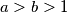$a > b > 1$ be relatively prime positive integers. Define the weight of an integer$c$, denoted by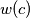$w(c)$ to be the minimal possible value of$|x| + |y|$ taken over all pairs of integers$x$ and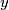$y$ such that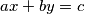$ax + by = c$. An integer$c$ is called a local champion if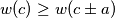$w(c) \geq w(c \pm a)$ and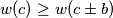$w(c) \geq w(c \pm b)$. Find all local champions and determine their number.
Izvor: Međunarodna matematička olimpijada, shortlist 2006